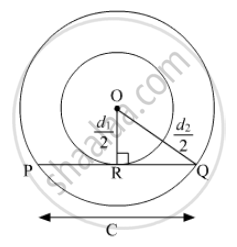Advertisement Remove all ads

# If D 1 , D 2 ( D 2 > D 1 ) Be the Diameters of Two Concentric Circle S and C Be the Length of a Chord of a Circle Which is Tangent to the Other Circle , Prove that D 2 2 = C 2 + D 1 2 . - Mathematics

Short Note

If $d_1 , d_2 ( d_2 > d_1 )$ be the diameters of two concentric circle s and c be the length of a chord of a circle which is tangent to the other circle , prove that${d_2}^2 = c^2 + {d_1}^2$.

Advertisement Remove all ads

#### SolutionLet O be the centre of two concentric circles and PQ be the tangent to the inner circle that touches the circle at R.

Now, OQ= $\frac{1}{2} d_2$   and

OR=$\frac{1}{2} d_1$

Also, PQ = c

As, PQ is the tangent to the circle.

⇒ OR ⊥ PQ

⇒ QR =$\frac{1}{2}PQ = \frac{1}{2}c$

In Triangle OQR,

∴ By Pythagoras Theorem,

$\left( OQ \right)^2 = \left( OR \right)^2 + \left( RQ \right)^2$
$\Rightarrow \left( \frac{d_2}{2} \right)^2 = \left( \frac{d_1}{2} \right)^2 + \left( \frac{c}{2} \right)^2$
$\Rightarrow \left( d_2 \right)^2 = \left( d_1 \right)^2 + c^2$

Is there an error in this question or solution?
Advertisement Remove all ads

#### APPEARS IN

RD Sharma Class 10 Maths
Chapter 8 Circles
Exercise 8.2 | Q 30 | Page 37
Advertisement Remove all ads
Advertisement Remove all ads
Share
Notifications

View all notifications

Forgot password?